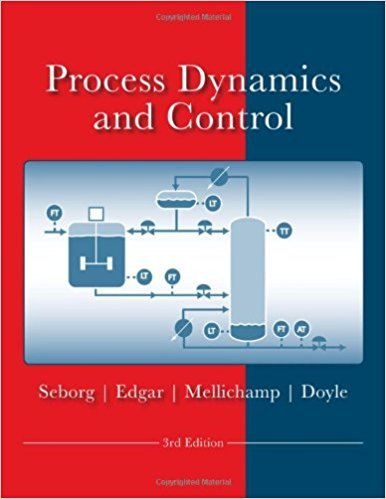×
Get Full Access to Process Dynamics And Control - 3 Edition - Chapter 18 - Problem 18.9
Get Full Access to Process Dynamics And Control - 3 Edition - Chapter 18 - Problem 18.9

×

# A binary distillation column has three tray temperatureISBN: 9780470128671 148

## Solution for problem 18.9 Chapter 18

Process Dynamics and Control | 3rd Edition

• Textbook Solutions
• 2901 Step-by-step solutions solved by professors and subject experts
• Get 24/7 help from StudySoup virtual teaching assistantsProcess Dynamics and Control | 3rd Edition

4 5 1 306 Reviews
23
0
Problem 18.9

A binary distillation column has three tray temperature measurements (17th, 24th, 30th trays) that can be used as possible controlled variables. Controlling temperature is equivalent to controlling composition. Step testing gives the following steady-state input-output relationships (u1 = steam pressure in reboiler; u2 = reflux ratio): Th = 1.5ul + 0.5uz (1) Tz4 = 2.0u1 + 1.7uz (2) T3o = 3.4ul + 2.9uz (3)All variables are deviation variables. Select the 2 X 2 control system that has the most desirable interactions, as determined by the RGA (note that there are three possible 2 X 2 control configurations). Explain why the combination of Tz4 and T3o is the least desirable controlled variable set, based on analyzing Eqs. (2) and (3) and the resulting determinant

Step-by-Step Solution:
Step 1 of 3

Uses of the Equations of Motion • Very few exact solutions of the full equations: – Unidirectional laminar flows are one class of exact solutions. – Simple shapes: spheres, ellipsoids, tori, etc., have solutions. – Most problems not amenable to exact analytical solutions. – Linear vs. nonlinear mathematics. • Dimensional analysis: – Insights even without solving the equations. – Scaling for length, velocity, pressure, etc. • Computational (numerical) solution: – Modern digital computers and the rise of CFD. – Commercial CFD software. Special team projects during the week of the AICHE Annual Meeting (Nov. 9-13) CHE377 – Fall 2016 Dimensionless Equations (BSL Ch. 3)

Step 2 of 3

Step 3 of 3

##### ISBN: 9780470128671

This full solution covers the following key subjects: variables, controlling, desirable, temperature, controlled. This expansive textbook survival guide covers 24 chapters, and 420 solutions. Since the solution to 18.9 from 18 chapter was answered, more than 499 students have viewed the full step-by-step answer. The full step-by-step solution to problem: 18.9 from chapter: 18 was answered by , our top Engineering and Tech solution expert on 11/15/17, 04:03PM. This textbook survival guide was created for the textbook: Process Dynamics and Control, edition: 3. Process Dynamics and Control was written by and is associated to the ISBN: 9780470128671. The answer to “A binary distillation column has three tray temperature measurements (17th, 24th, 30th trays) that can be used as possible controlled variables. Controlling temperature is equivalent to controlling composition. Step testing gives the following steady-state input-output relationships (u1 = steam pressure in reboiler; u2 = reflux ratio): Th = 1.5ul + 0.5uz (1) Tz4 = 2.0u1 + 1.7uz (2) T3o = 3.4ul + 2.9uz (3)All variables are deviation variables. Select the 2 X 2 control system that has the most desirable interactions, as determined by the RGA (note that there are three possible 2 X 2 control configurations). Explain why the combination of Tz4 and T3o is the least desirable controlled variable set, based on analyzing Eqs. (2) and (3) and the resulting determinant” is broken down into a number of easy to follow steps, and 123 words.

Unlock Textbook Solution